Miscellaneous

Chapter 7 Class 12 Integrals
Serial order wise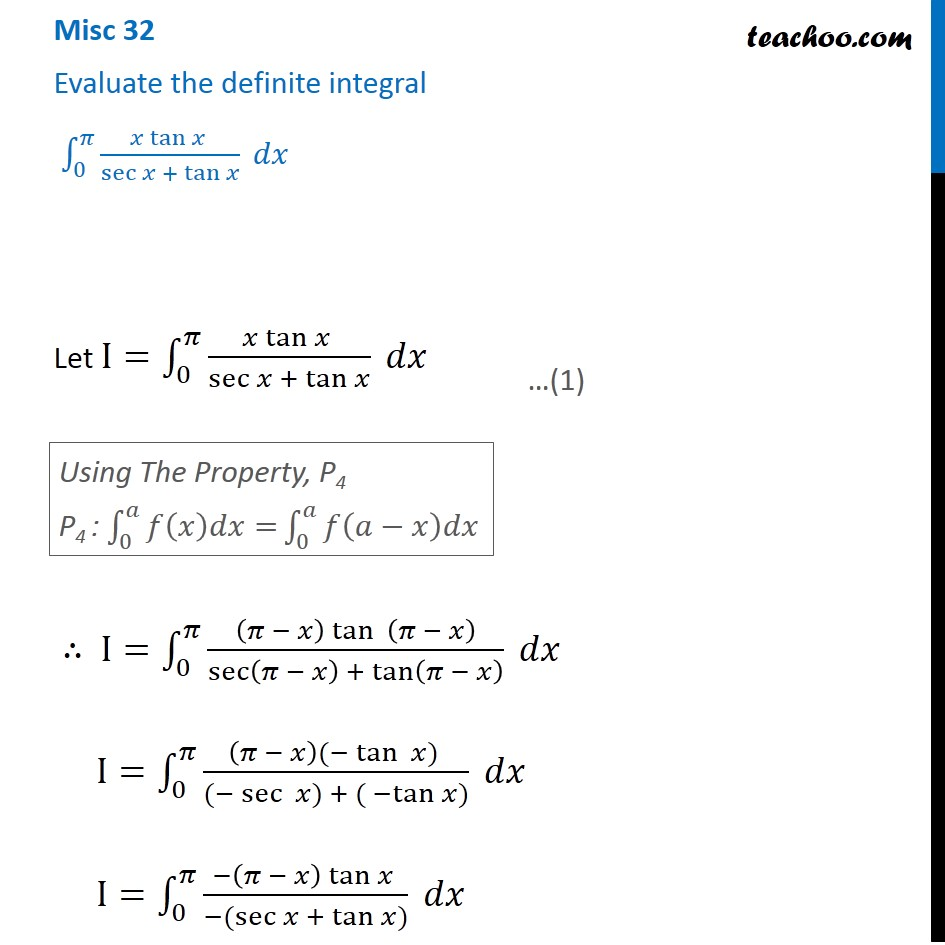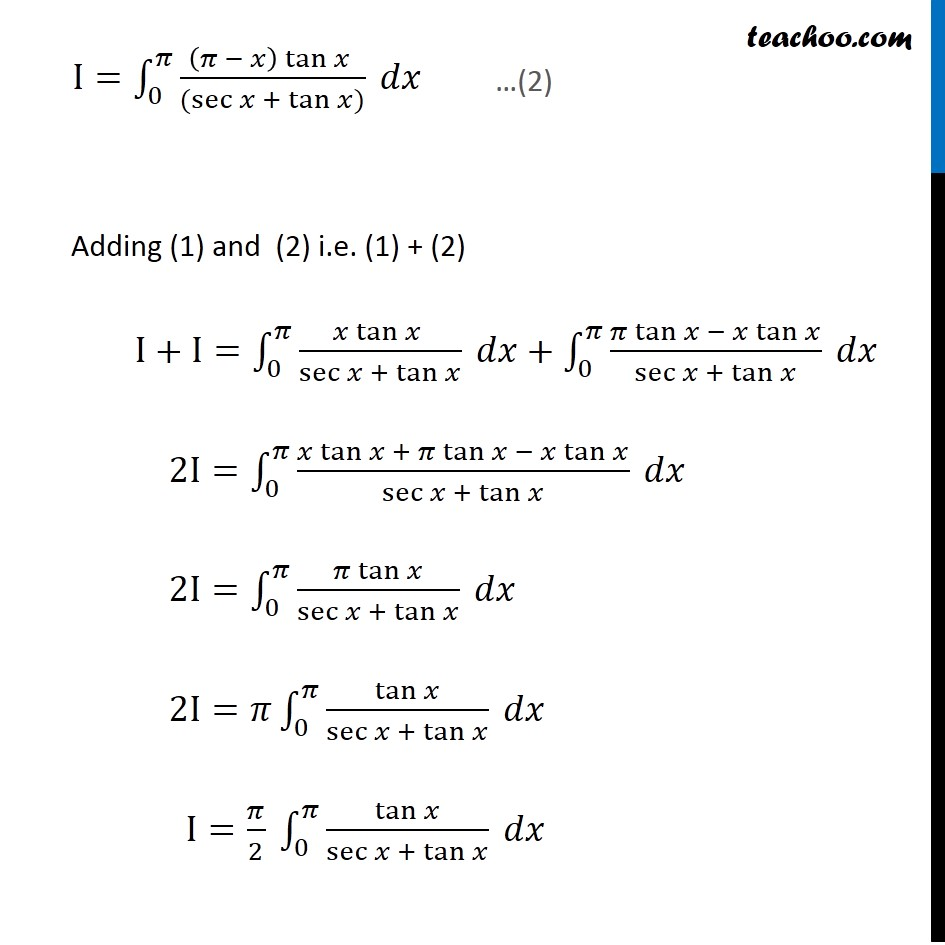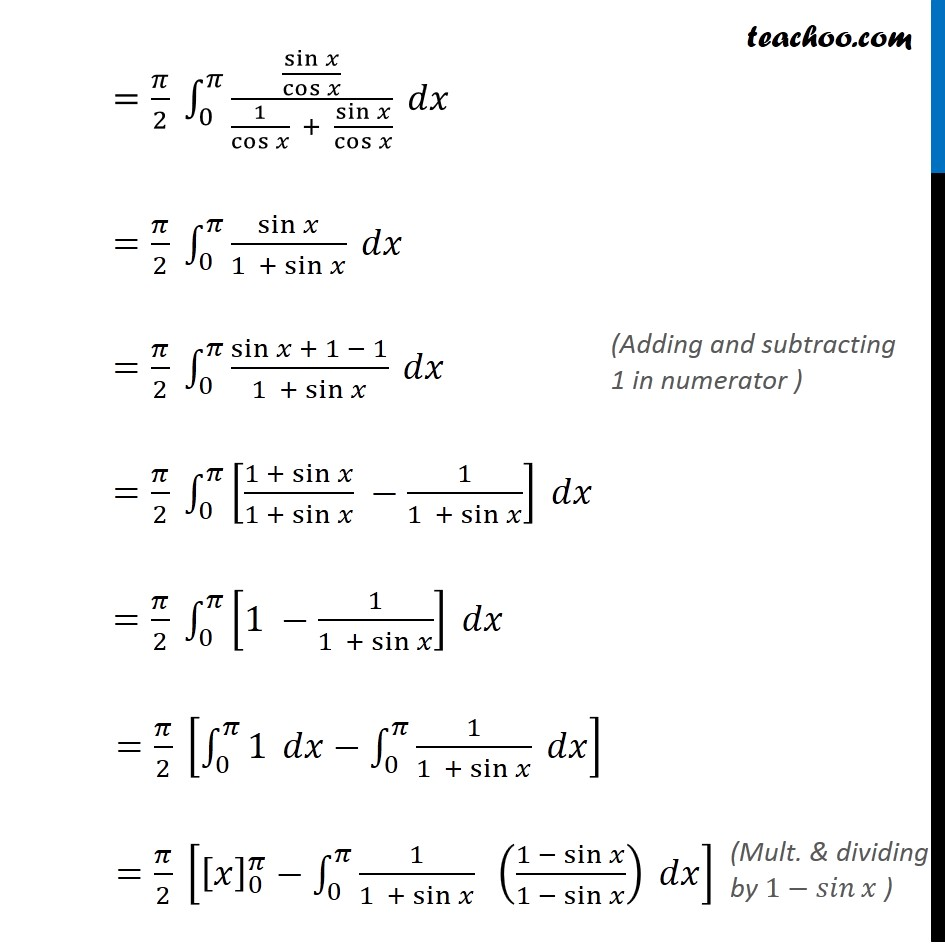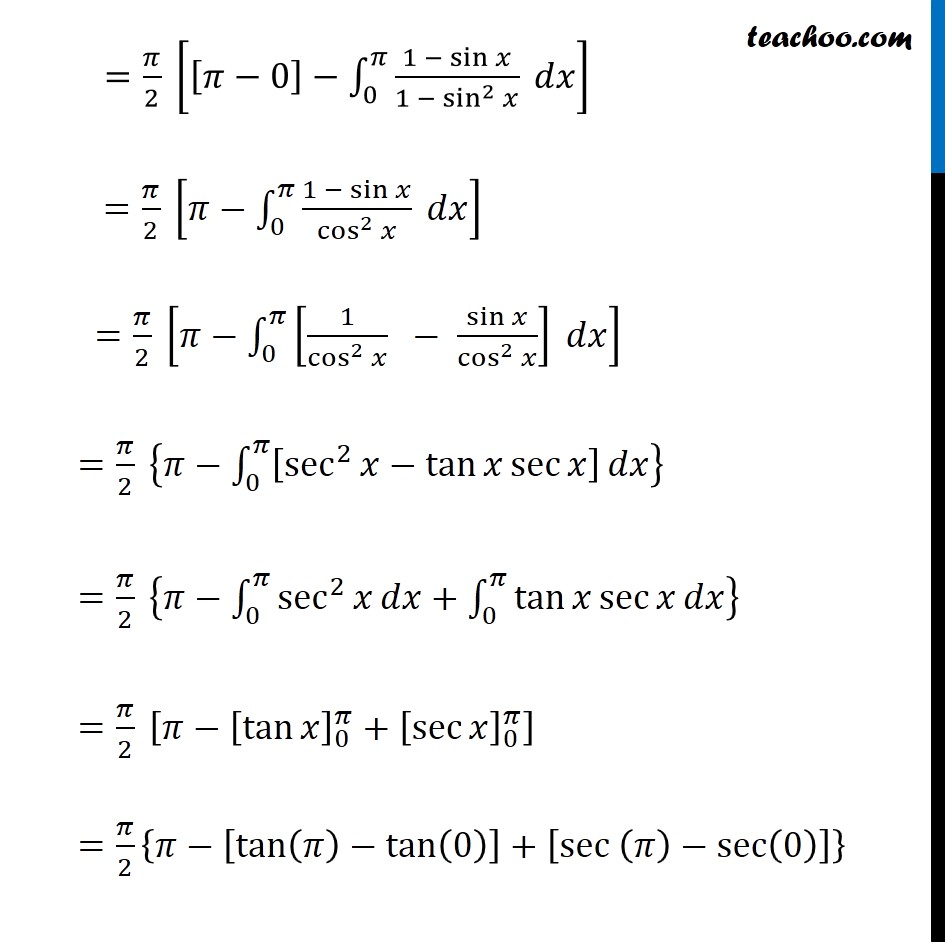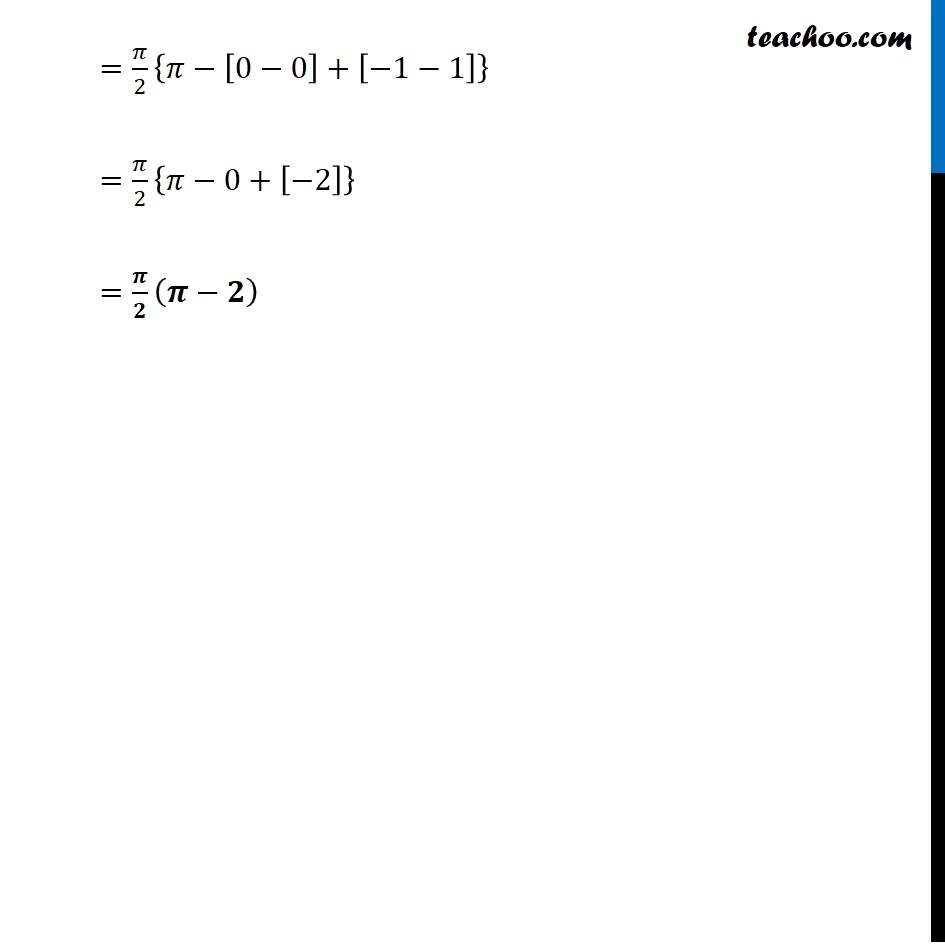Learn in your speed, with individual attention - Teachoo Maths 1-on-1 Class

### Transcript

Question 2 Evaluate the definite integral ∫_0^𝜋▒(𝑥 tan⁡𝑥 )/(sec⁡𝑥 +〖 tan〗⁡𝑥 ) 𝑑𝑥 Let I=∫_0^𝜋▒(𝑥 tan⁡𝑥 )/(sec⁡𝑥 +〖 tan〗⁡𝑥 ) 𝑑𝑥 ∴ I=∫_0^𝜋▒((𝜋 − 𝑥) tan⁡〖 (𝜋 − 𝑥)〗)/(sec⁡(𝜋 − 𝑥) +〖 tan〗⁡(𝜋 − 𝑥) ) 𝑑𝑥 I=∫_0^𝜋▒((𝜋 − 𝑥)(−tan⁡〖 𝑥〗) )/((−sec⁡〖 𝑥〗) + 〖( −tan〗⁡𝑥)) 𝑑𝑥 I=∫_0^𝜋▒(−(𝜋 − 𝑥) tan⁡𝑥 )/(−(sec⁡𝑥 +〖 tan〗⁡𝑥)) 𝑑𝑥 Using The Property, P4 P4 : ∫_0^𝑎▒〖𝑓(𝑥)𝑑𝑥=〗 ∫_0^𝑎▒𝑓(𝑎−𝑥)𝑑𝑥 I=∫_0^𝜋▒((𝜋 − 𝑥) tan⁡𝑥 )/((sec⁡𝑥 +〖 tan〗⁡𝑥)) 𝑑𝑥 Adding (1) and (2) i.e. (1) + (2) I+I=∫_0^𝜋▒(𝑥 tan⁡𝑥 )/(sec⁡𝑥 +〖 tan〗⁡𝑥 ) 𝑑𝑥+∫_0^𝜋▒(𝜋 tan⁡𝑥 − 𝑥 tan⁡𝑥)/(sec⁡𝑥 +〖 tan〗⁡𝑥 ) 𝑑𝑥 2I=∫_0^𝜋▒(𝑥 tan⁡𝑥 + 𝜋 tan⁡𝑥 − 𝑥 tan⁡𝑥)/(sec⁡𝑥 +〖 tan〗⁡𝑥 ) 𝑑𝑥 2I=∫_0^𝜋▒(𝜋 tan⁡𝑥)/(sec⁡𝑥 +〖 tan〗⁡𝑥 ) 𝑑𝑥 2I=𝜋∫_0^𝜋▒tan⁡𝑥/(sec⁡𝑥 +〖 tan〗⁡𝑥 ) 𝑑𝑥 I=𝜋/2 ∫_0^𝜋▒tan⁡𝑥/(sec⁡𝑥 +〖 tan〗⁡𝑥 ) 𝑑𝑥 =𝜋/2 ∫_0^𝜋▒(sin⁡𝑥/cos⁡𝑥 )/(1/cos⁡𝑥 + sin⁡𝑥/cos⁡𝑥 ) 𝑑𝑥 =𝜋/2 ∫_0^𝜋▒sin⁡𝑥/(1 + sin⁡𝑥 ) 𝑑𝑥 =𝜋/2 ∫_0^𝜋▒(sin⁡𝑥 + 1 − 1)/(1 + sin⁡𝑥 ) 𝑑𝑥 =𝜋/2 ∫_0^𝜋▒[(1 + sin⁡𝑥)/(1 + sin⁡𝑥 ) −1/(1 + sin⁡𝑥 )] 𝑑𝑥 =𝜋/2 ∫_0^𝜋▒[1 −1/(1 + sin⁡𝑥 )] 𝑑𝑥 =𝜋/2 [∫_0^𝜋▒1 𝑑𝑥−∫_0^𝜋▒1/(1 + sin⁡𝑥 ) 𝑑𝑥] =𝜋/2 [[𝑥]_0^𝜋−∫_0^𝜋▒1/(1 + sin⁡𝑥 ) ((1 − sin⁡𝑥)/(1 − sin⁡𝑥 )) 𝑑𝑥] =𝜋/2 [[𝜋−0]−∫_0^𝜋▒(1 − sin⁡𝑥)/(1 − sin^2⁡𝑥 ) 𝑑𝑥] =𝜋/2 [𝜋−∫_0^𝜋▒(1 − sin⁡𝑥)/cos^2⁡𝑥 𝑑𝑥] =𝜋/2 [𝜋−∫_0^𝜋▒[1/cos^2⁡𝑥 − sin⁡𝑥/cos^2⁡𝑥 ] 𝑑𝑥] =𝜋/2 {𝜋−∫_0^𝜋▒[sec^2⁡𝑥−tan⁡𝑥 sec⁡𝑥 ] 𝑑𝑥} =𝜋/2 {𝜋−∫_0^𝜋▒sec^2⁡𝑥 𝑑𝑥+∫_0^𝜋▒〖tan⁡𝑥 sec⁡𝑥 〗 𝑑𝑥} =𝜋/2 [𝜋−[tan⁡𝑥 ]_0^𝜋+[sec⁡𝑥 ]_0^𝜋 ] =𝜋/2 {𝜋−[tan⁡〖(𝜋)−tan⁡(0) 〗 ]+[sec (𝜋)−sec⁡(0) ]} =𝜋/2 {𝜋−[0−0]+[−1−1]} =𝜋/2 {𝜋−0+[−2]} =𝝅/𝟐 (𝝅−𝟐)